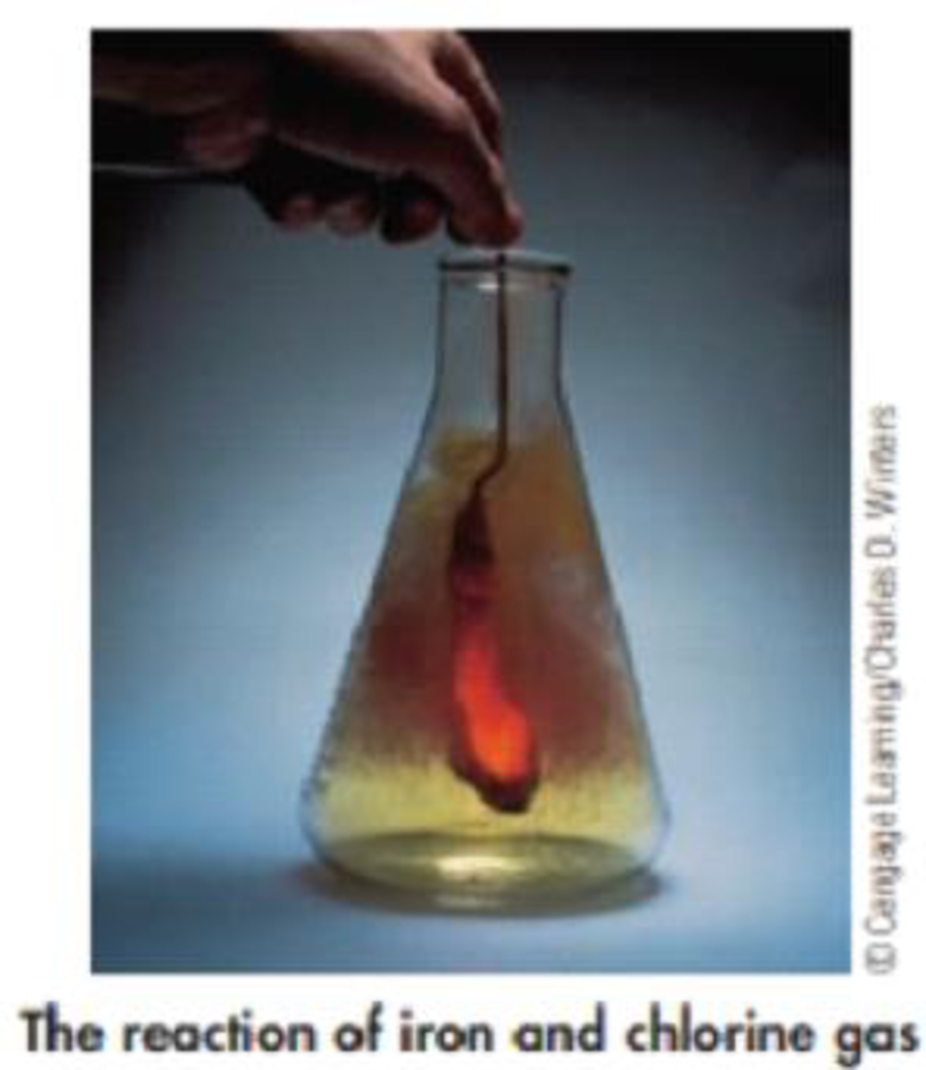Chapter 4, Problem 82GQ

Chapter
Section
Textbook Problem

The reaction of iron metal and chlorine gas to give iron(III) chloride is illustrated below.(a) Write the balanced chemical equation for the reaction.(b) Beginning with 10.0 g of iron, what mass of Cl2, in grams, is required for complete reaction? What mass of FeCl3 can be produced?(c) If only 18.5 g of FeCl3 is obtained from 10.0 g of iron and excess Cl2, what is the percent yield?(d) If 10.0 g each of iron and chlorine are combined, what is the theoretical yield of iron(III) chloride?

(a)

Interpretation Introduction

Interpretation:

Balanced chemical equation for the reaction of iron metal with chlorine gas forming iron(III) chloride has to be written.

Concept introduction:

• For chemical reaction balanced chemical reaction equation written in accordance with the Law of conservation of mass.
• Law of conservation of mass states that for a reaction total mass of the reactant and product must be equal.
Explanation

The balanced chemical reaction equation for any reaction is written in accordance with the law of conservation of mass.

Here iron metal is reacting with chlorine gas to give iron (III) chloride.

The balanced chemical reaction equation for this reaction is,

2Fe(s)+3

(b)

Interpretation Introduction

Interpretation:

The mass of chlorine required to complete the reaction with 10.0g of Fe has to be determined.  Mass of FeCl3 produced in the reaction has to be calculated.

Concept Introduction:

• The molar mass of an element or compound is the mass in grams of 1 mole of that substance, and it is expressed in the unit of grams per mol (g/mol).
• Product of a chemical reaction is the produced compounds or the compounds formed after a chemical reaction.
• Stoichiometric factor is a relationship between reactant and product which is obtained from the balanced chemical equation for a particular reaction.

(c)

Interpretation Introduction

Interpretation:

The percent yield of FeCl3 has to be determined when 18.5 g of FeCl3 is obtained from 10.0 g of iron and excess of Cl2.

Concept Introduction:

• Percentageyield=ActualyieldTheoreticalyield×100
• Theoretical yield is the maximum product yield that can be expected based on the masses of the reactants and the reaction stoichiometry.

(d)

Interpretation Introduction

Interpretation:

The theoretical yield of FeCl3 when 10.0g of iron and 10.0g of chlorine combined has to be determined.

Concept Introduction:

Limiting reagent: limiting reagent is a reactant, which consumes completely in the chemical reaction. The quantity of the product depends on this limiting reagent, it can be determined with the help of balanced chemical equation for the reaction.

Still sussing out bartleby?

Check out a sample textbook solution.

See a sample solution

The Solution to Your Study Problems

Bartleby provides explanations to thousands of textbook problems written by our experts, many with advanced degrees!

Get Started

Organic nutrients include all of the following except. a. minerals b. fat c. carbohydrates d. protein

Nutrition: Concepts and Controversies - Standalone book (MindTap Course List)

2.47 What is a metalloid?

Chemistry for Engineering Students

___ causes the pupil to widen. a. Low light b. Bright light

Biology: The Unity and Diversity of Life (MindTap Course List)